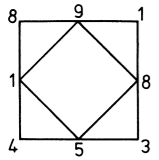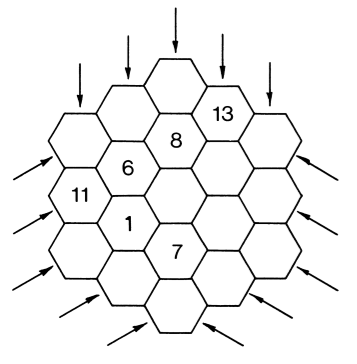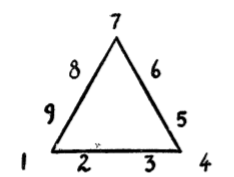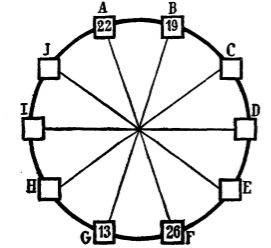## 1. Take-away number squares

### Begin with four numbers, one number at each corner of the square.### Draw a square around the previous one and make sure you go though the corners. At each corner of the larger square put the difference of the numbers at the next-door-corner of the small square.## 2. a magic honeycomb

### Using the numbers 1 through 19,  make each bee cell row add up to 38.## 3. Squares Around a Triangle

### Arrange numbers 1 – 9 along the sides and corners of an equilateral triangle so that the sums of the squares of the four numbers along each side of the triangle are equal.## 4. the ten-spoke wheel

### For example. 222 + 192 = 132 + 262